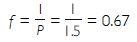# NCERT Solutions for Class 10 Science Chapter 10 - Light Reflection and Refraction

##### Question 1:

Define the principal focus of concave mirror.

The principal focus (PF) is a point on the principal axis of a concave mirror where the rays parallel to principal axis meet after reflection from the mirror.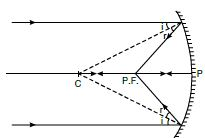##### Question 2:

The radius of curvature of a spherical mirror is 20 cm. What is its focal length?

For convex mirror, R = +20 cm and R = 2f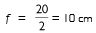For concave mirror, R = – 20 cm and R = 2f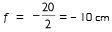##### Question 3:

Name a mirror which can give an erect and enlarged image of an object.

A concave mirror produces an erect and enlarged image when the object is placed between the pole and focus as shown in the figure.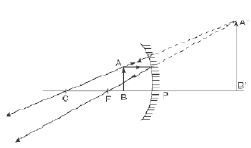##### Question 4:

Why do we prefer a convex mirror as a back-view mirror in vehicles?

For the same size of the mirrors (plane and convex), the field of view of plane mirror is from A to B [Figure (a)], whereas the field of view of convex mirror is much larger, i.e., from A´ to B´ [Figure (b)].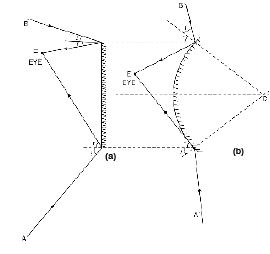##### Question 5:

Find the focal length of a convex mirror whose radius of curvature is 32 cm.?

Radius of curvature, R = 32 cm

We know that, focal length =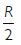Therefore, f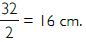##### Question 6:

A concave mirror produces three time magnified (enlarged) real image of an object placed at 10 cm in front of it. Where is the image located?

Linear magnification, m = –3 [real image is always inverted]
u = –10 cm [u is always negative]
As m = –v/u
v = –mu
v = –(–3) (–10)
v = –30 cm
Thus, the real image is formed at 30 cm in front of the mirror (on the same side as the object).

##### Question 7:

A ray of light travelling in air enters obliquely into water. Does the light ray bend towards normal or away from normal? Why?

The ray is travelling from an optically rarer medium (air) to an optically denser medium (water); therefore, it bends towards the normal.
Also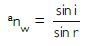##### Question 8:

Light enters from air to glass having a refractive index of 1.50. What happens to the speed of light in glass? Given: Speed of light in vacuum is 3 × 108 ms–1.

Refractive index of glass, n = 1.50
Speed of light in vacuum, c = 3 ×108 m/s,
Speed of light in glass, v = c/n
= 3 × 108/1.5 = 2 ×108 ms–1

##### Question 9:

A concave mirror produces three time magnified (enlarged) real image of an object placed at 10 cm in front of it. Where is the image located?

Linear magnification, m = –3 [real image is always inverted]
u = –10 cm [u is always negative]
As m = –v/u
v = –mu
v = –(–3) (–10)
v = –30 cm
Thus, the real image is formed at 30 cm in front of the mirror (on the same side as the object).

##### Question 10:

A ray of light travelling in air enters obliquely into water. Does the light ray bend towards normal or away from normal? Why?

The ray is travelling from an optically rarer medium (air) to an optically denser medium (water); therefore, it bends towards the normal.
Also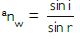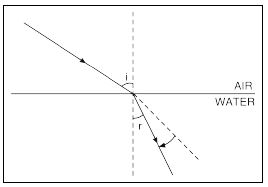##### Question 11:

What are the different ways in which glucose is oxidised to provide energy in various organisms?

Different pathways to provide energy from glucose are depicted in the following chart: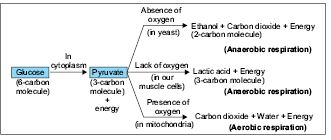##### Question 12:

Light enters from air to glass having a refractive index of 1.50. What happens to the speed of light in glass? Given: Speed of light in vacuum is 3 × 108 ms–1.

Refractive index of glass, n = 1.50
Speed of light in vacuum, c = 3 × 108 m/s,
Speed of light in glass, v = c/n
= 3 × 108/1.5 = 2 × 108 ms–1

##### Question 13:

Find out from the following table the medium having the highest optical density. Also, find the medium with the lowest optical density.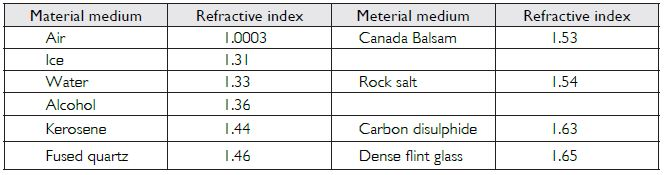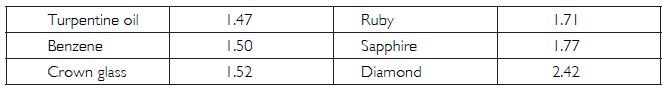Optical density depends upon refractive index. The higher the refractive index, the higher the optical density and vice versa. Diamond has the highest optical density, as it has the highest refractive index (n = 2.42). And, due to lowest refractive index of air (n = 1.0003), it has the lowest optical density.

##### Question 14:

You are given kerosene, turpentine oil and water. In which of these, does the light travel fastest? Use the table given in the above question.

Light travels the fastest in a medium whose refractive index is smallest i.e., in water (n = 1.33)

##### Question 15:

The refractive index of diamond is 2.42. What is the meaning of this statement?

Refractive index in diamond =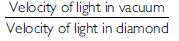\ Velocity of light in diamond =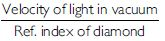The statement means that velocity of light in diamond is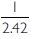times the velocity of light in vacuum. i.e.,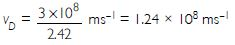##### Question 16:

Define 1 dioptre of power of a lens.

Power of a lens is the reciprocal of focal length. 1 dioptre is the power of a lens having focal length 1 metre.

##### Question 17:

How are water and minerals transported in plants?

Water and minerals are transported in plants by xylem and phloem tissues in the following ways: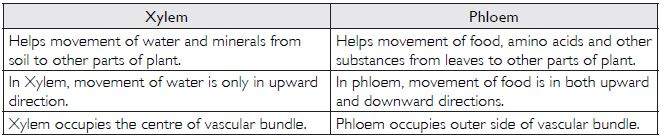##### Question 18:

A convex lens forms a real and inverted image of a needle at a distance of 50 cm from it. Where should be the needle placed in front of the convex lens if the image is equal to size of the object? Also, find the power of the lens.

A convex lens forms a real, inverted image of the size of the object if the object is placed at centre of curvature i.e., at 2f. In this case.
u = –50 cm, v = m × 50 cm; m = –1 \ v = 50 cm; and 2f = 50 cm or f = 25 cm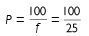or P = 4 dioptre.

##### Question 19:

Find the power of a concave lens of focal length 2 m.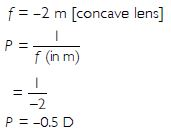##### Question 20:

Which of the following materials cannot be used to make a lens?

1. Water
2. Glass
3. Plastic
4. Clay

Clay since it is opaque. The material of a lens must be transparent.

##### Question 21:

The image formed by a concave mirror is observed to be virtual, erect and larger than object. Where should be the position of the object?

1. Between principal focus and the centre of curvature
2. At centre of curvature
3. Beyond centre of curvature
4. Between the pole of the mirror and its principal focus

Between the pole of the mirror and its principal focus

##### Question 22:

Where should be an object placed in front of a convex lens to get a real image of the size of the object?

1. At principal focus of the lens
2. At twice the focal length of lens
3. At infinity
4. Between optical centre of the lens and its principal focus

At twice the focal length of the lens

##### Question 23:

A spherical mirror and a thin spherical lens have each a focal length of –15 cm. The mirror and lens are likely to be:

1. Both are concave
2. Both are convex
3. Mirror is concave and lens is convex
4. Mirror is convex but lens is concave

Both are concave

##### Question 24:

No matter how far you stand from a mirror, your image appears erect. The mirror is likely to be

1. only plane
2. only concave
3. only convex
4. either plane or convex

Either plane or convex. Both give erect images, where size of image in plane mirror is same but it is diminished in convex mirror.

##### Question 25:

Which of the following lenses would you prefer to use while reading small letters in a dictionary?

1. A convex lens of focal length 50 cm
2. A concave lens of focal length 50 cm
3. A convex lens of focal length 5 cm
4. A concave lens of focal length 5 cm/li>

A convex lens of focal length 5 cm.
Since, a convex lens gives a magnified image of the object and smaller the focal length, more the magnifying power.

##### Question 26:

We wish to obtain an erect image of an object, using a concave mirror of focal length 15 cm. What should be the range of distance of the object from the mirror? What is the nature of the image? Is the image larger or smaller than the object? Draw a ray diagram to show the image formation in this case.

When the object is placed between the pole and principal focus of the concave mirror, an erect, enlarged, virtual image is formed behind the mirror. Therefore, the range of distance is greater than zero and less than focal length (which is less than 15 cm from mirror).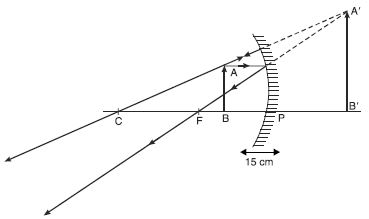##### Question 27:

Name the type of mirror used in the following situations:
(b) Side/rear-view mirror of a vehicle
(c) Solar furnace

(a) Concave mirrors are used for headlights of the car to give concentrated beam of light.
(b) Side/rear-view mirror of a vehicle is mostly convex because it gives wider field of view, which enables a driver to see most of the traffic behind.
(c) Solar furnace uses concave mirror to concentrate heat to a point.

##### Question 28:

One half of a convex lens is covered with a black paper. Will this lens produce a complete image of the object? Explain your observation.

Convex lens will form a complete image even if is covered with a black paper but the intensity (brightness) of image will half as that with complete lens exposed. Nature, size and location of the image will be the same since light from all parts of the object reach the exposed part of the lens.
It can be understood by the following two cases:
1. When the upper half of the lens is covered:
In this case, the ray of light coming from the object will be refracted by the lower half of the lens. These rays meet at the other side of the lens to form the image of the given object.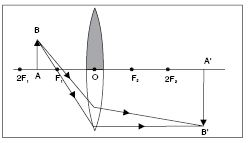2. When the lower half of the lens is covered:
In this case, the ray of light coming from the object will be refracted by the upper half of the lens. These rays meet at the other side of the lens to form the image of the given object.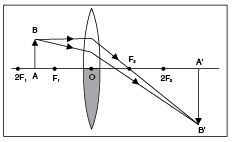##### Question 29:

An object 5 cm in length is held 25 cm away from a converging lens of focal length 10 cm. Draw the ray diagram and find the position, size and the nature of image formed.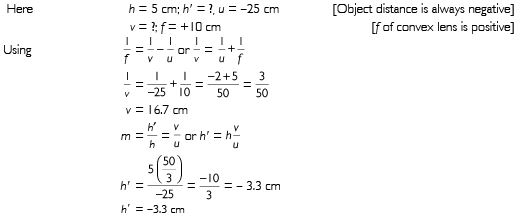Negative sign shows image is inverted, real, diminished (3.3 cm) and at 16.7 cm on the right side of lens.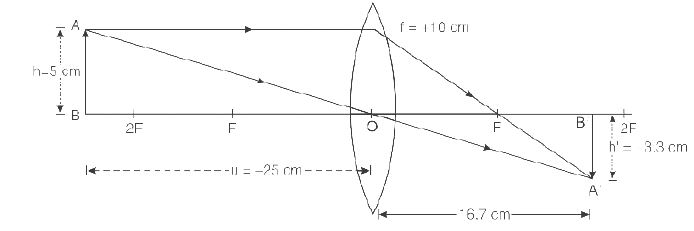##### Question 30:

A concave lens of focal length 15 cm forms an image 10 cm from the lens. How far is the object placed from the lens? Draw the ray diagram.

u = ? ; v = –10 cm [real image]
f = –15 cm [f of concave lens is negative];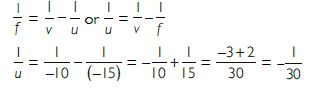u = –30 cm
Negative sign shows that the object is placed to the left of lens.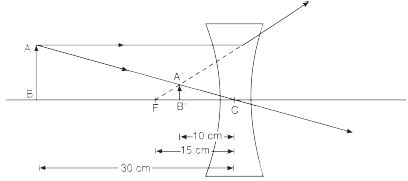##### Question 31:

An object is placed at a distance of 10 cm from a convex mirror of focal length 15 cm. Find the position and nature of the image.

u = –10 cm [u is always negative] ;
f = +15 cm [convex mirror]
v = ?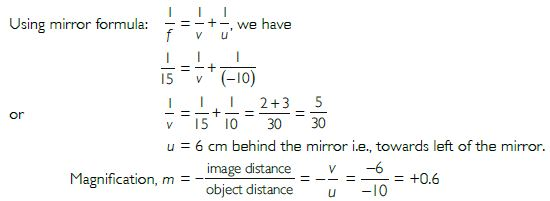Positive value of magnification ⇒ Image is virtual and erect.

.
##### Question 32:

The magnification produced by a plane mirror is +1. What does this mean?

u = –10 cm [u is always negative] ;
f = +15 cm [convex mirror]
v = ?Positive value of magnification ⇒ Image is virtual and erect.

##### Question 33:

The magnification produced by a plane mirror is +1. What does this mean?

It means that image produced by plane mirror is erect and of the same size as of the object. The image is virtual too.

##### Question 34:

An object 5.0 cm in length is placed at a distance of 20 cm in front of a convex mirror of radius of curvature 30 cm. Find the position, nature and size of the image.

h = 5.0 cm; u = –20 cm [u is always negative];
r = +30 cm; [convex mirror: R positive]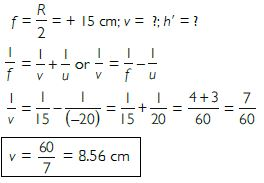Positive value of v ⇒ image is formed behind the mirror or to the right of mirror.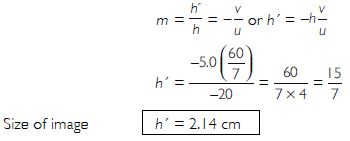Positive value of image height ⇒ image formed is erect and virtual. Moreover, it is smaller in size.

##### Question 35:

An object of size 7.0 cm is placed at 27 cm in front of a concave mirror of focal length 18 cm. At what distance from the mirror should the screen be placed, so that a sharp focused image can be obtained? Find the size and nature of the image.

h = 7.0 cm; u = –27 cm [u is always negative];
f = –18 cm [f of concave mirror is negative]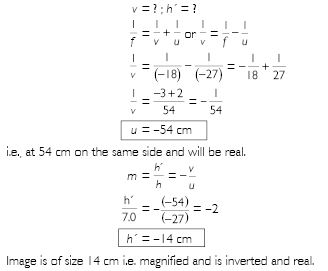##### Question 36:

Find the focal length of a lens of power –2.0 D. What type of lens is this?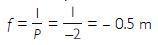Therefore, f = – 0.5 m = – 50 cm
Negative power of the lens indicates that the lens must be concave.

##### Question 37:

A doctor has prescribed a corrective lens of power +1.5 D. Find the focal length of lens. Is the prescribed le